##### Question

In: Statistics and Probability

# Salaries for teachers in a particular elementary school district have a mean of $44,000 and a... Salaries for teachers in a particular elementary school district have a mean of$44,000 and a standard deviation of $6,500. We randomly survey 36 teachers from that district. 1. Why can we say the sampling distribution of mean salaries for teachers in this district is approximately normal? 2. Find the probability that the mean salary is less than$43,000.
3. Find the probability that the mean salary is between $45,000 and$47,000.

## Solutions

##### Expert Solution

Solution :

Given that ,

mean =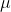= 44000

standard deviation =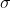= 6500

n = 36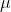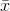= 44000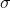=/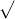n = 6500 /36  = 1083.3333

The sampling distribution of mean= 44000

The standard deviation of the sampling mean= 1083.3333

P(< 43000 ) = P((-) /< ( 43000 - 44000 ) / 1083.3333 )

= P(z < -0.92)

Using z table

= 0.1788

Probability = 0.1788

P( 45000 << 47000 )

= P[( 45000 - 44000) / < (-) /< ( 47000 - 44000) / 1083.3333 )]

= P(0.92 < Z < 2.77 )

= P(Z < 2.77 ) - P(Z < 0.92 )

Using z table,

= 0.9972 - 0.8212

= 0.1760

Probability = 0.1760

## Related Solutions

##### Salaries for teachers in a particular elementary school district are normally distributed with a mean of...
Salaries for teachers in a particular elementary school district are normally distributed with a mean of $44,000 and a standard deviation of$6,500. We randomly survey ten teachers from that district. 1.Find the probability that the teachers earn a total of over $400,000 2.If we surveyed 70 teachers instead of ten, graphically, how would that change the distribution in part d? 3.If each of the 70 teachers received a$3,000 raise, graphically, how would that change the distribution in part...
##### Salaries for teachers in a particular elementary school district are normally distributed with a mean of...
Salaries for teachers in a particular elementary school district are normally distributed with a mean of $44,000 and a standard deviation of$6,500. We randomly survey ten teachers from that district. Find the 85th percentile for the sum of the sampled teacher's salaries to 2 decimal places.
##### A researcher claims that the mean of the salaries of elementary school teachers is greater than...
A researcher claims that the mean of the salaries of elementary school teachers is greater than the mean of the salaries of secondary school teachers in a large school district. The mean of the salaries of a random sample of 26 elementary school teachers is $48,250 and the sample standard deviation is$3900. The mean of the salaries of 24 randomly selected secondary school teachers is $45,630 with a sample standard deviation of$5530. At ? = 0.05, can it...
##### A study was conducted to estimate the difference in the mean salaries of elementary school teachers...
A study was conducted to estimate the difference in the mean salaries of elementary school teachers from two neighboring states. A sample of 10 teachers from the Indiana had a mean salary of $28,900 with a standard deviation of$2300. A sample of 14 teachers from Michigan had a mean salary of $30,300 with a standard deviation of$2100. Determine a 95% confidence interval for the difference between the mean salary in Indiana and Michigan.(Assume population variances are different.) *Include...
##### A researcher claims that the variation in the salaries of elementary school teachers is greater than...
A researcher claims that the variation in the salaries of elementary school teachers is greater than the variation in the salaries of secondary school teachers. A random sample of the salaries of 30 elementary school teachers has a variance of $8,208, and a random sample of the salaries of 30 secondary school teachers has a variance of$3,817. At α = 0.05, can the researcher conclude that the variation in the elementary school teachers’ salaries is greater than the variation...
##### A study was conducted to determine if the salaries of elementary school teachers from two neighboring...
A study was conducted to determine if the salaries of elementary school teachers from two neighboring districts were equal. A sample of 15 teachers from each district was randomly selected. The mean from the first district was $28,900 with a standard deviation of$2300. The mean from the second district was $30,300 with a standard deviation of$2100. Assume the samples are random, independent, and come from populations that are normally distributed. Construct a 95% confidence interval for μ1 -...